# Velocity Formula

Velocity Formula

Velocity is a measure of how quickly an object moves. So, the velocity is the change in the position of an object, divided by the time. Velocity has a magnitude (a value) and a direction. The unit for velocity is meters per second (m/s).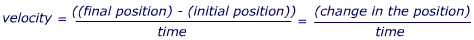v = velocity (m/s)

xf = the final position (m)

xi = the initial position (m)

t = the time in which the change occurs (s)

Δx = short form for "the change in" position (m)

Velocity Formula Questions:

1) A sail boat is in a 1000 m race, and it crosses the starting line when it is already at full speed. It reaches the finish line in exactly 1 minute and 20 seconds ( = 80.0 s). What is the velocity of the sail boat?

Answer: The initial position is the starting line, which we can give the value xi = 0.00 m. The finish line is 1000 m from the start, so xf = 1000 m. The time it takes the sail boat to travel that distance is t = 80.0 s. The velocity can be found using the equation: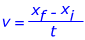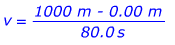v = 12.5 m/s

The velocity is 12.5 m/s, in the direction of the finish line.

2) Each floor in a tall building is 3.00 m high. When it's moving, the elevator in this building moves at a constant velocity of 1.50 m/s. If the first floor is at position 0.00 m, the second floor is at position 3.00 m, and so on, how much time does it take for the elevator to go from the sixth (6th) to the eighteenth (18th) floor?

Answer: The initial and final positions of the elevator can be found using the floor numbers and the distance between floors. The initial floor is 6, so the initial position is:

xi = (6)(3.00 m)

xi = 18.0 m

and the final floor is 18, so the final position is:

xf = (18)(3.00 m)

xf = 54.0 m

The velocity (which we assume to be constant) is v = 1.50 m/s. The time must be found, so rearrange the equation: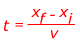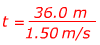t = 24.0 s

The time it takes for the elevator to travel from the sixth to the eighteenth floor is 24.0 seconds.

 Related Links: Velocity Practice Problems Quiz Speed and Velocity Quiz